# Cumulative DI For Bank PO Exam

• Last Updated : 15 Jul, 2022

Importance of CUMULATIVE DI.

In Banking exams such as IBPS, SBI, RRB PO, and Clerk, CUMULATIVE DI plays an important role in the Quantitative aptitude section. In prelims or mains exams, there are high chances you will get at least one set including 5 questions from a CUMULATIVE DI. Aspirants should practice questions on CUMULATIVE DI.

Direction (1-5): Read the following information carefully and answer the questions given below.

The given bar graph shows the cumulative percentage distribution of the total number of visitors to Agra and Delhi together on five different days i.e. Monday, Tuesday, Wednesday, Thursday, and Friday and the given table chart shows the cumulative number of visitors to Agra in the five days.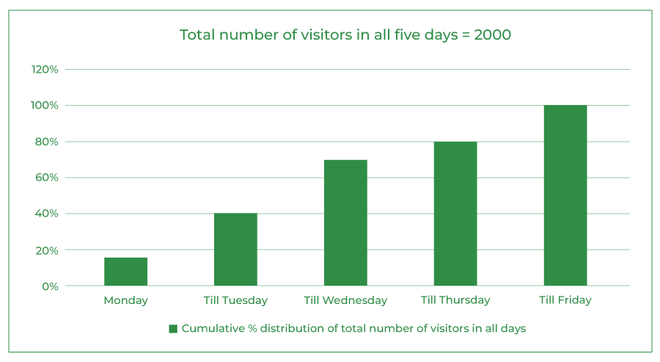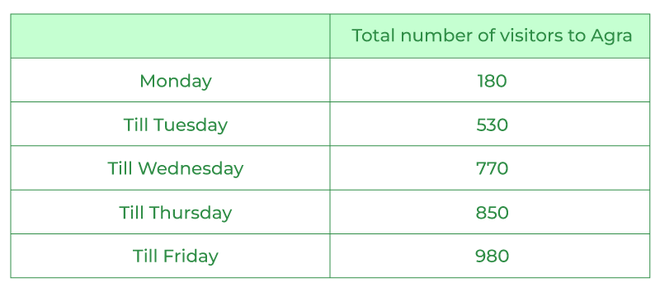Que 1. The total number of visitors to Delhi on Monday and Wednesday together is what percentage of the total number of visitors to Agra on Wednesday?
A) 200%
B) 150%
C) 125%
D) 120%
E) None of these

Que 2. Find the average total number of visitors to Delhi on all days except Monday.
A) 280
B) 260
C) 245
D) 225
E) None of these

Que 3. If 20% of the visitors to Agra on Tuesday are foreigners and 40% of the visitors to Delhi on Friday are foreigners, then find the total number of visitors to Agra on Tuesday who are not foreigners and the total number of visitors to Delhi on Friday are foreigners.
A) 460
B) 388
C) 492
D) 422
E) None of these

Que 4. Find the difference between the total number of visitors to Delhi on Wednesday and the average number of visitors to Agra on Monday and Thursday.
A) 180
B) 230
C) 150
D) 190
E) None of these

Que 5. Find the sum of the average total number of visitors to Agra and Delhi on Tuesday and the total number of visitors to Agra on Thursday.
A) 330
B) 430
C) 350
D) 380
E) None of these

Direction (6-10): Read the following information carefully and answer the questions given below.

The given table shows the cumulative percentage of the total number of flowers (Oriental Lilies and Asiatic White Lilies) sold by florist L on four different days namely Monday, Tuesday, Wednesday, and Thursday, and also shows the cumulative number of Oriental lilies sold by florist L on four different days.

Total number of flowers sold by L = 700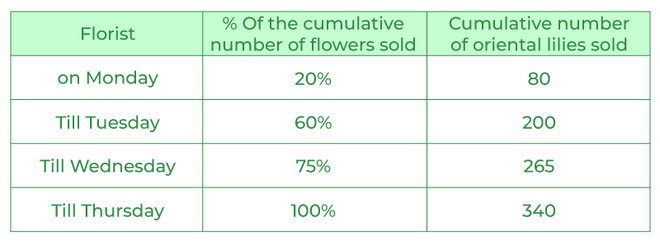Que 6. Find the ratio between the total number of Oriental lilies sold on Wednesday and Thursday together and the total number of Asiatic white lilies sold by Thursday.
A) 4:3
B) 7:5
C) 5:2
D) 2:3
E) None of these

Que 7. The total number of Asiatic white lilies sold on Tuesday and Wednesday is how much more than the total number of Oriental lilies sold on Tuesday.
A) 80
B) 125
C) 70
D) 105
E) None of these

Que 8. Find the sum of the average of the total number of Asiatic white lilies sold on Tuesday, Wednesday and Thursday and the total number of Oriental lilies sold on Monday.
A) 250
B) 225
C) 180
D) 200
E) None of these

Que 9. The total number of Asiatic white lilies sold on Monday is what percentage of the total number of Oriental lilies sold on Thursday?
A) 80%
B) 75%
C) 50%
D) 45%
E) None of these

Que 10. If the total number of flowers sold on Friday is 20% more than the total number of flowers sold on Thursday and the ratio of Oriental lilies sold on Friday and Asiatic white lilies sold on Friday is 7:3, then find the total number of Oriental lilies sold on Friday.
A) 187
B) 167
C) 130
D) 147
E) None of these

Direction (11-15): Read the following information carefully and answer the questions given below.

The given table shows the cumulative percentage distribution of the total number of students who attended the exam (Physics and Maths) and scored marks in five different categories and the cumulative number of students who attended the Physics exam and scored marks in five categories. Each student attended only one exam i.e., either the Physics exam or the Maths exam.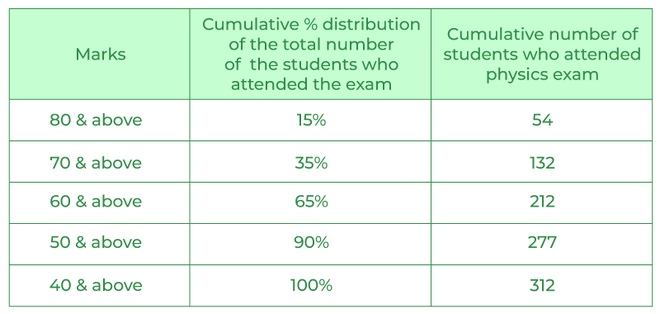Note:-
The total number of students who are attended the exams is 600.
No one failed in the exam.

Que 11. Find the sum of the total number of students who scored more than 70 marks but less than 80 marks in the Physics exam and the total number of students who scored more than 80 marks in Maths.

A) 165
B) 114
C) 136
D) 142
E) None of these

Que 12. Find the ratio of the total number of students who scored more than 50 marks but less than 60 marks in Physics to the total number of students who scored more than 40 marks but less than 50 marks in maths exam.
A) 12:11
B) 8:7
C) 3:4
D) 13:5
E) None of these

Que 13. The number of students who scored 80 and above marks in Physics is what percentage of the number of students who scored above 60 marks but less than 70 in Maths?
A) 54%
B) 60%
C) 75%
D) 45%
E) None of these

Que 14. Find the difference between the total number of students who scored more than 70 but less than 80 marks in Maths and the total number of students who scored more than 40 but less than 50 in Physics.
A) 10
B) 8
C) 7
D) 15
E) None of these

Que 15. Find the average of the total number of students who scored more than 50 marks but less than 60 marks in Maths and the total number of students who scored more than 50 marks but less than 60 marks in Physics.
A) 90
B) 75
C) 100
D) 70
E) None of these

Directions (16-20): Read the following information carefully and answer the questions.
The given line graph shows the percentage distribution of the number of black cars sold out of the total number of cars (black and white) sold and the given table chart shows the cumulative percentage distribution of the total number of cars sold in five different months i.e. May, June, July, August and September.

Total number of cars sold in all 5 months =1200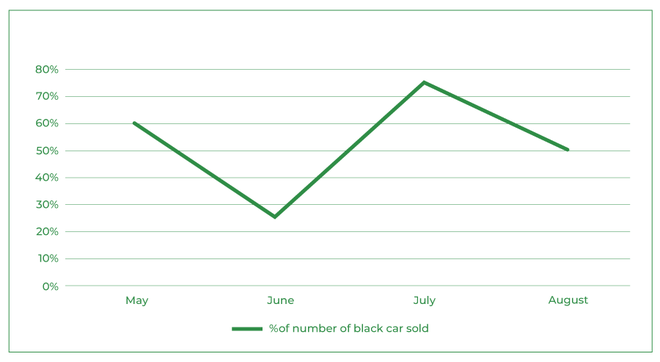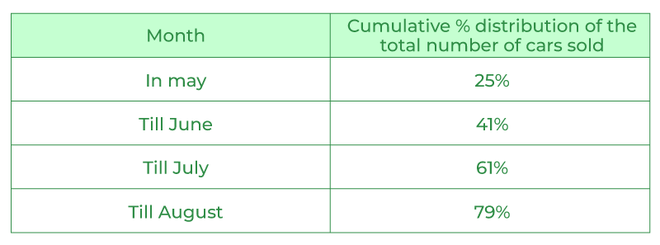Que 16. What is the total number of black cars sold in August?
A) 90
B) 100
C) 80
D) 108
E) None of these

Que 17. What is the difference between the number of white cars sold in June and the number of black cars sold in August?
A) 60
B) 45
C) 52
D) 36
E) None of these

Que 18. Find the average number of white cars sold in May, June and July together?
A) 135
B) 112
C) 108
D) 154
E) None of these

Que 19. If the number of white cars sold in September is 126 and then the number of black cars sold in September is how much percentage more than the number of white cars sold in May?
A) 10%
B) 5%
C) 20%
D) 15%
E) None of these

Que 20. Find the ratio of the total number of cars sold in May and the average number of black and white cars sold in August?
A) 11:7
B) 9:4
C) 25:9
D) 17:12
E) None of these

Direction (1-5):

% of total number of visitors to Agra and Delhi together on Monday = 15%
% of total number of visitors to Agra and Delhi together on Tuesday = 40% – 15% = 25%
% of total number of visitors to Agra and Delhi together on Wednesday = 70% – 40% = 30%
% of total number of visitors to Agra and Delhi together on Thursday = 80% – 70% = 10%
% of total number of visitors to Agra and Delhi together on Friday = 100% – 80% = 20%
Total number of visitors to Agra and Delhi together on Monday = 2000 * 15/100 = 300
Total number of visitors to Agra and Delhi together on Tuesday = 2000 * 25/100 = 500
Total number of visitors to Agra and Delhi together on Wednesday = 2000 * 30/100 = 600
Total number of visitors to Agra and Delhi together on Thursday = 2000 * 10/100 = 200
Total number of visitors to Agra and Delhi together on Friday = 2000 * 20/100 = 400
Total number of visitors to Agra on Monday = 180
Total number of visitors to Agra on Tuesday = 530 – 180 = 350
Total number of visitors to Agra on Wednesday = 770 – 530 = 240
Total number of visitors to Agra on Thursday = 850 – 770 = 80
Total number of visitors to Agra on Friday = 980 – 850 = 130
Total number of visitors to Delhi on Monday = 300 – 180 = 120
Total number of visitors to Delhi on Tuesday = 500 – 350 = 150
Total number of visitors to Delhi on Wednesday = 600 – 240 = 360
Total number of visitors to Delhi on Thursday = 200 – 80 = 120
Total number of visitors to Delhi on Friday = 400– 130 = 270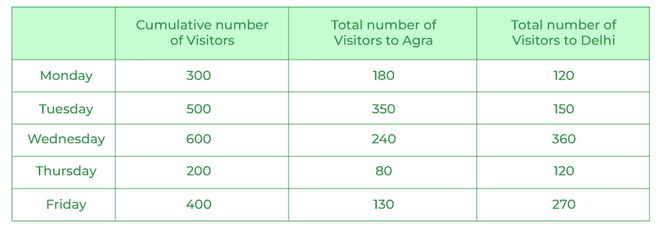Required percentage = (120 + 360)/240 * 100 = 200%

Required average = (150 + 360 + 120 + 270)/4 = 225

Total number of visitors to Agra on Tuesday who are not foreigners = 350 * 80/100 = 280
Total number of visitors to Delhi on Friday are foreigners = 270 * 40/100 = 108
Required total = 280 + 108 = 388

Required difference = 360 – (180 + 80)/2 = 230

Required sum = 500/2 + 80 = 330

Direction (6-10):
% of total number of flowers sold on Monday = 20%
% of total number of flowers sold on Tuesday = 60% – 20% = 40%
% of total number of flowers sold on Wednesday = 75% – 60% = 15%
% of total number of flowers sold on Thursday= 100% – 75% = 25%
Total number of Oriental lilies sold on Monday = 80
Total number of Oriental lilies sold on Tuesday = 200 – 80 = 120
Total number of Oriental lilies sold on Wednesday = 265 – 200 = 65
Total number of Oriental lilies sold on Thursday = 340 – 265 = 75
Total number of flowers sold on Monday = 700 * 20/100 = 140
Total number of flowers sold on Tuesday = 700 * 40/100 = 280
Total number of flowers sold by on Wednesday = 700 * 15/100 = 105
Total number of flowers sold on Thursday = 700 * 25/100 = 175
Total number of Asiatic white lilies sold on Monday = 140 – 80 = 60
Total number of Asiatic white lilies sold on Tuesday = 280 – 120 = 160
Total number of Asiatic white lilies sold on Wednesday = 105 – 65 = 40
Total number of Asiatic white lilies sold on Thursday = 175 – 75 = 100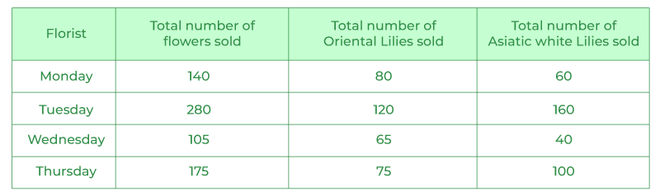Required ratio = (65 + 75) : 100 = 7 : 5

Total number of Asiatic white lilies sold on Tuesday and Wednesday = 200
Total number of Oriental lilies sold on Tuesday= 120
Required difference = 200 – 120 = 80

Required sum = (160 + 40 + 100)/3 + 80 = 100 + 80 = 180

Required percentage = 60/75 * 100 = 80%

Total number of flowers sold on Friday = 175 + 175 * 20/100 = 210
Total number of Oriental lilies sold on Friday = 210 * 7/10 = 147

Direction (11-15):
% of total number of the students attending the exams and scored above 80 marks = 15%
% of total number of the students attending the exams and scored between 70 and 79 = 35% -15% = 20%
% of total number of the students attending the exams and scored between 60 and 69 = 65% – 35% = 30%
% of total number of the students attending the exams and scored between 50 and 59 = 90% – 65% = 25%
% of total number of the students attending the exams and scored between 40 and 49 = 100% -90% = 10%
Total number of the students attending the exams and scored above 80 marks = 600 * 15/100 = 90
Total number of the students attending the exams and scored between 70 and 79 = 600 * 20/100 = 120
Total number of the students attending the exams and scored between 60 and 69 = 600 * 30/100 = 180
Total number of the students attending the exams and scored between 50 and 59 = 600 * 25/100 = 150
Total number of the students attending the exams and scored between 40 and 49 = 600 * 10/100 = 60
Total number of students who scored above 80 marks in Physics = 54
Total number of students who scored between 70 -79 marks in Physics = 132 – 54 = 78
Total number of students who scored between 60 – 69 marks in Physics = 212 – 132 = 80
Total number of students who scored between 50 – 59 marks in Physics = 277 – 212 = 65
Total number of students who scored between 40 – 49 marks in Physics = 312 – 277 = 35
Total number of students who scored above 80 marks in Maths = 90 – 54 = 36
Total number of students who scored between 70 -79 marks in Maths = 120 – 78 = 42
Total number of students who scored between 60 – 69 marks in Maths = 180 – 80 = 100
Total number of students who scored between 50 – 59 marks in Maths = 150 – 65 = 85
Total number of students who scored between 40 – 49 marks in Maths = 60 – 35 = 25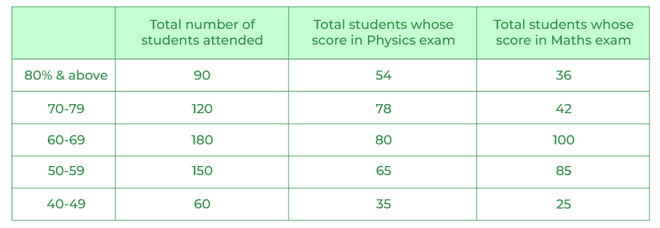Total students who scored more than 70 but less than 80 in physics = 78

The total number of students who scored more than 80 in maths = 36
Required sum = 78 + 36 = 114

Required ratio = 65 : 25 = 13 : 5

Required percentage = 54/100  * 100 = 54%

Required difference = 42 – 35 = 7

Required average = (85 + 65)/2 = 75

Directions (16-20):
Total number of cars sold in May =1200 * 25/100 = 300
Number of black cars sold in May =300 * 60/100 = 180
Number of white cars sold in May = 300 – 180 = 120
% of the total number of cars sold in June = 41 – 25 = 16%
Total number of cars sold in June = 1200 * 16/100=192
Number of black cars sold in June = 192 * 25/100=48
Number of white cars sold in June = 192 – 48 = 144
% of the total number of cars sold in July = 61 – 41 = 20%
Total number of cars sold in July = 1200 * 20/100 = 240
Number of black cars sold in July =240 * 75/100 = 180
Number of white cars sold in July =240 – 180 = 60
% of the total number of cars sold in August =79 – 61 = 18%
Total number of cars sold in August =1200 * 18/100 = 216
Number of black cars sold in August =216 * 50/100 = 108
Number of white cars sold in August = 216 – 108 = 108
Total number of cars sold in September = 1200-(300+192+240+216)=252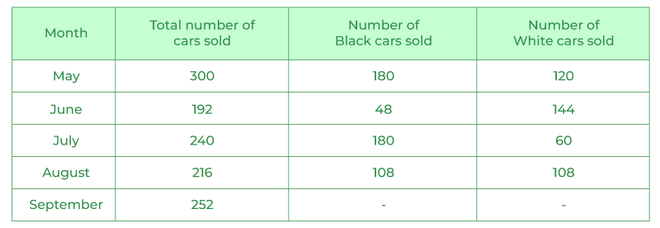The total number of black cars sold in August = 108 cars

Required difference=144 – 108=36

Required average=(120 + 144 + 60)/3 = 324/3 = 108

Number of white cars sold in September = 126
Number of black cars sold in September = 126
Required percentage=(126 – 120)/120 × 100 = 6/120 × 100 = 5%